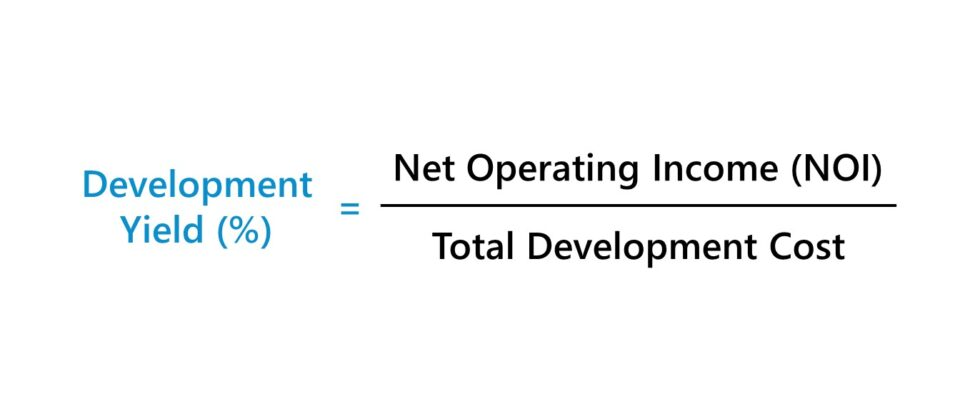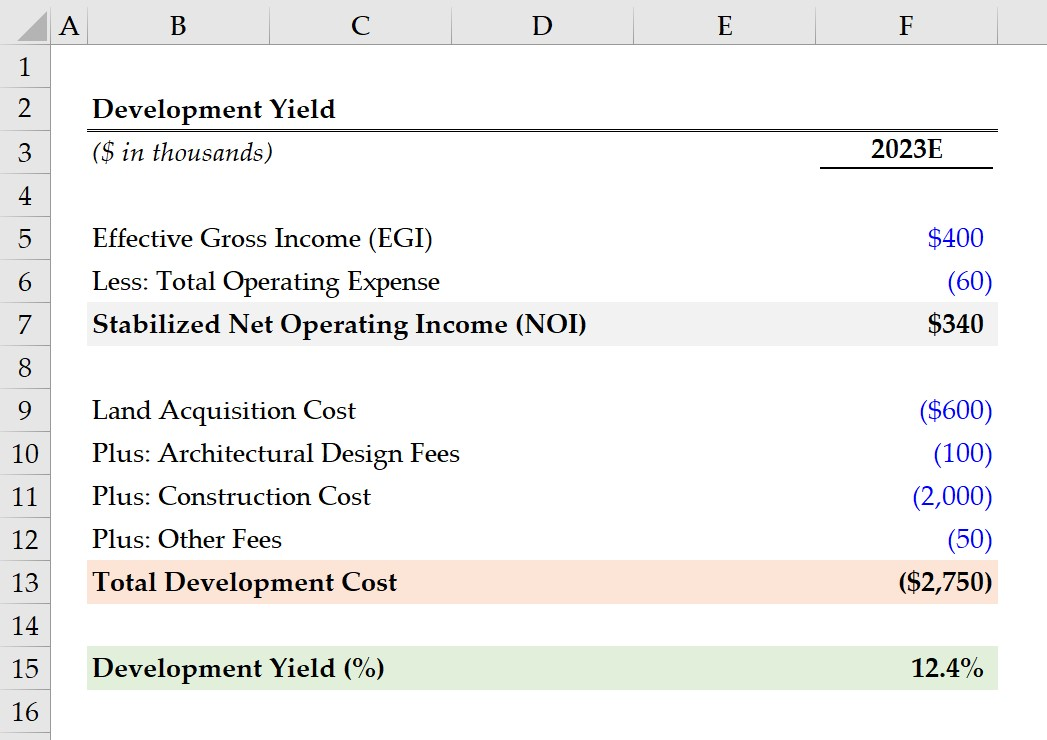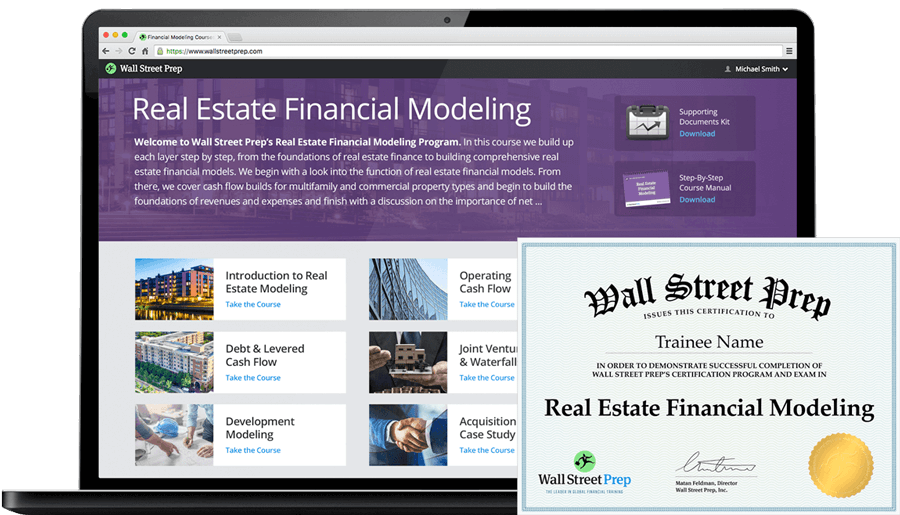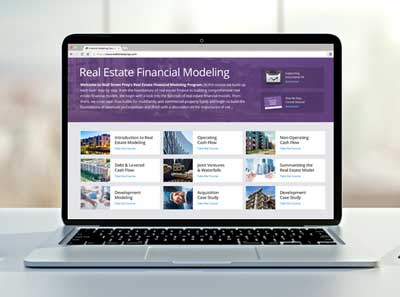Welcome to Wall Street Prep! Use code at checkout for 15% off.# Development Yield

Guide to Understanding the Development Yield## How to Calculate Development Yield?

In the context of real estate investing and property development, the development yield measures the potential return on investment (ROI) earned on a property development project.

While most often used for development projects – i.e. when purchasing land to design and contract a new property – the development yield can still be used for property improvement projects, such as value-add acquisitions, albeit to a lesser extent.

Conceptually, the development yield is the potential return on investment (ROI) generated on a property investment.

Once determined, the implied development yield on a property investment is then compared to the yield on comparable investments to gauge the financial viability of the project, i.e. confirm the implied return is enough for the investor, given the risk profile.

The development yield on a property investment can be calculated using the following steps:

1. Calculate Annual Net Operating Income (NOI)
2. Divide Annual NOI by Total Development Cost
3. Convert to Percentage Form (Multiply by 100)

By calculating the development yield – which is a relatively quick process (i.e. “back of the envelope” calculation) – real estate investors and property developers can compare the yield on different projects and analyze the risks and rewards of each to make informed decisions around which projects to pursue.## Development Yield Formula

The development yield formula is the ratio between annual net operating income (NOI) and total development cost.

Development Yield (%) = Stabilized Net Operating Income (NOI) ÷ Total Development Cost

Where:

• Annual Net Operating Income (NOI) → The numerator, net operating income (NOI), represents the annual profits generated by the property after deducting operating expenses, such as property management costs, maintenance costs, property taxes, and insurance fees. The NOI used here is on a post-stabilization basis, i.e. the property is developed and fully operational (and thus generating income).
• Total Development Cost → The denominator, the total development cost, consists of the land acquisition cost (i.e. the purchase price of the land in which the development will occur), the development costs (i.e. all the incurred costs from planning, designing, and constructing the project, including architect fees, construction materials, labor costs, and other operating expenses). The composition of the total development cost is directly tied to the project type, i.e. a development project vs. a purchase of an existing property with minimal improvements.

Note, the development yield is most often expressed as a percentage, so the resulting figure in decimal form must then be converted into percentage form by multiplying by 100.

## What is a Good Development Yield?

There is no set benchmark for what constitutes a “good” development yield, as that would be contingent on the investment type. Per usual, a riskier investment must generate higher returns to compensate the investor for the additional risk undertaken, otherwise there is no economic incentive.

A couple of other factors that must be considered include the property’s location (i.e. urban, suburban, rural, etc.), the risk tolerance of the investor, and the expected time horizon before the property reaches a state of stabilization.

There are also external factors such as the current conditions of the real estate market (supply/demand dynamics) and the state of the economy (e.g. interest rate environment and outlook) that can influence an investor’s decision.

Generally speaking, however, a higher development yield is perceived to be more attractive to a real estate investor, all else being equal.

Each developer will set their target yield based on appetite for risk and strategy to meet their targeted return on the investment, so performing due diligence and market research to ensure the development yield on the particular project aligns with their investment criteria tends to take precedence over the development yield.

## Development Yield vs. Cap Rate: What is the Difference?

The capitalization rate (or “cap rate”) and yield on cost (YoC) are other return metrics usually used by real estate investors to analyze property investments.

At initial glance, the formula of the two metrics are similar in that both are ratios, with net operating income (NOI) acting as the numerator.

However, the difference between the cap rate and development yield lies in the denominator.

The cap rate is the ratio between the annual NOI and the fair market value (FMV) of the property, whereas the development yield is the ratio between the stabilized NOI and total development cost.

Therefore, the development yield concept can be thought of as the “forward looking” cap rate, since the timing in which the development yield is measured is most often before any design or construction occurs, i.e. the property has no appraised fair value yet.

In practice, there is another metric measured by real estate investors termed the “development spread”, which is the difference between the development yield (i.e. the “going-in” cap rate) and the market cap rate (i.e. the “going-out” cap rate).

## Development Yield Calculator

We’ll now move on to a modeling exercise, which you can access by filling out the form below.Submitting...

## Development Yield Calculation Example

Suppose you are a real estate developer considering undertaking a long-term development project, where land will be purchased in 2023 to construct a commercial building.

Once the property is stabilized, effective gross income (EGI) is expected to be \$400,000, with total operating expenses projected to be \$60,000 per year.

• Effective Gross Income (EGI) = \$400,000
• Total Operating Expenses = \$60,000

The effective gross income (EGI) is the total potential revenue generated by a rental property, net of any vacancy and credit losses, while operating expenses include costs such as general & administrative costs, property management costs, and property taxes, among others.

By subtracting the annual gross rental income – i.e. the income earned from renting out the building to tenants – from the total operating expenses associated with managing the building, we can determine the stabilized annual net operating income (NOI) is \$340k.

• Stabilized Net Operating Income (NOI) = \$400,000 – \$60,000 = \$340,000

The cost of acquiring the land is \$500k, while the architectural design fees, construction costs, and other fees (e.g. permit approval, initial origination fee for loan application processing) are \$100k, \$1.5 million and \$200k, respectively.

• Land Acquisition Cost = \$600,000
• Architectural Design Fees = \$100,000
• Construction Cost = \$2 million
• Other Fees = \$50k

The sum of all costs is the total development cost, which comes out to \$2.75 million.

• Total Development Cost = \$600,000 + \$100,000 + \$2,000,000 + \$50,000 = \$2,750,000

In conclusion, we arrive at an implied development yield of 12.4% for our hypothetical property development project, which we calculated by dividing the building property’s annual NOI by its total development cost.

• Development Yield (%) = \$340,000 ÷ \$2,750,000 = 12.4%20+ Hours of Online Video Training

### Master Real Estate Financial Modeling

This program breaks down everything you need to build and interpret real estate finance models. Used at the world's leading real estate private equity firms and academic institutions.

Inline Feedbacks